# RD Sharma Solutions For Class 7 Maths Chapter - 6 Exponents

Chapter 6 – Exponents of RD Sharma Solutions for Class 7 are provided here. Students can get the pdf of this chapter from the given links. The syllabus given by the CBSE for all the classes is completely based on the RD Sharma Solutions. Class 7 is a stage where several new topics are introduced. BYJU’S RD Sharma Solutions Class 7 solutions for maths has been designed to help the students solve the CBSE class 7 Maths problems with ease.

Chapter 6 – Exponents, contains 4 Exercises. RD Sharma Solutions for Class 7 are given here contain the answers to all the questions present in these exercises. Let us have a look at some of the concepts that are being discussed in this chapter.

• Definition and meaning of exponents
• Finding the value of a number given in the exponential form
• Expressing the numbers in exponential form
• Laws of exponents
• Use of exponents in expressing large numbers in standard form
• Expanded exponential form

## Download the PDF of RD Sharma Solutions For Class 7 Maths Chapter 6 Exponents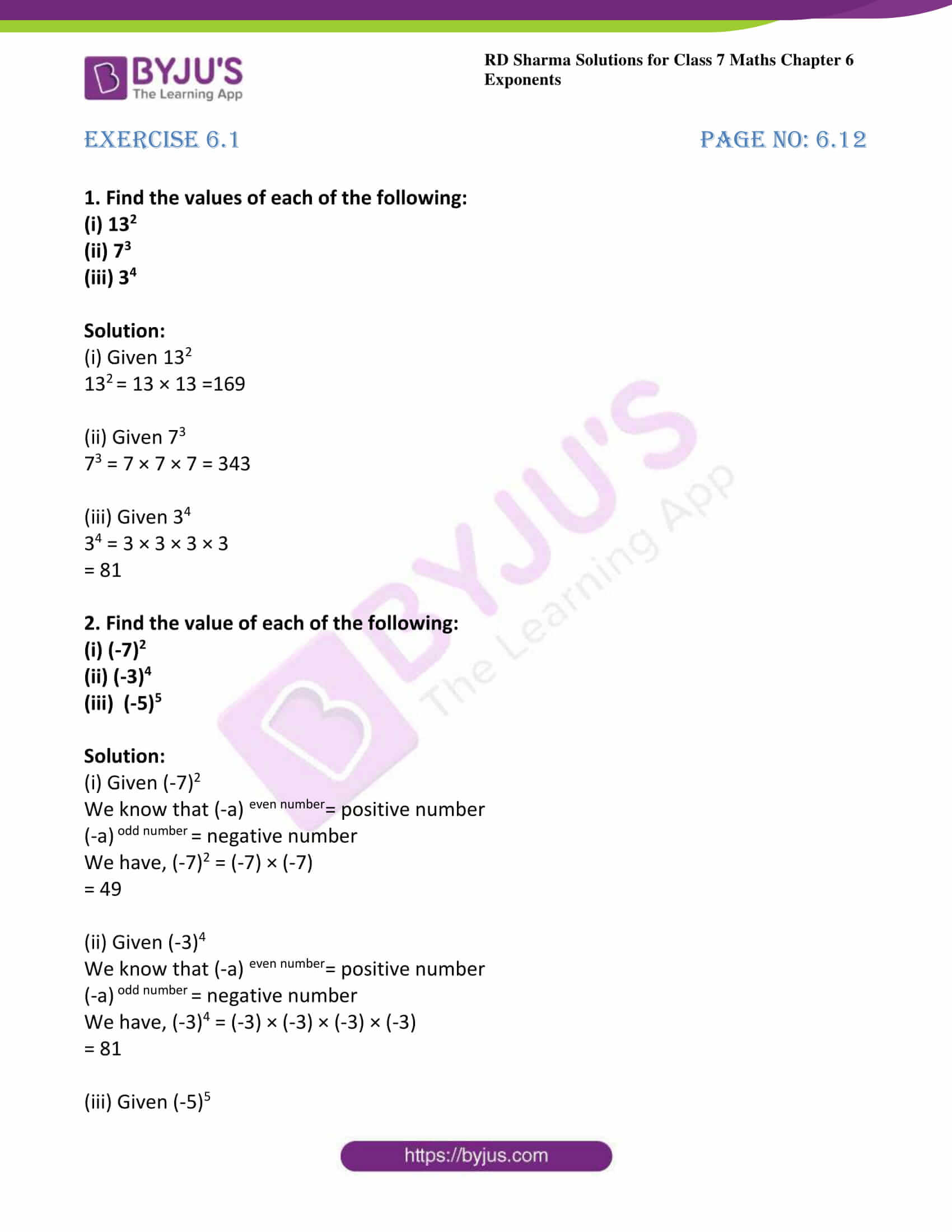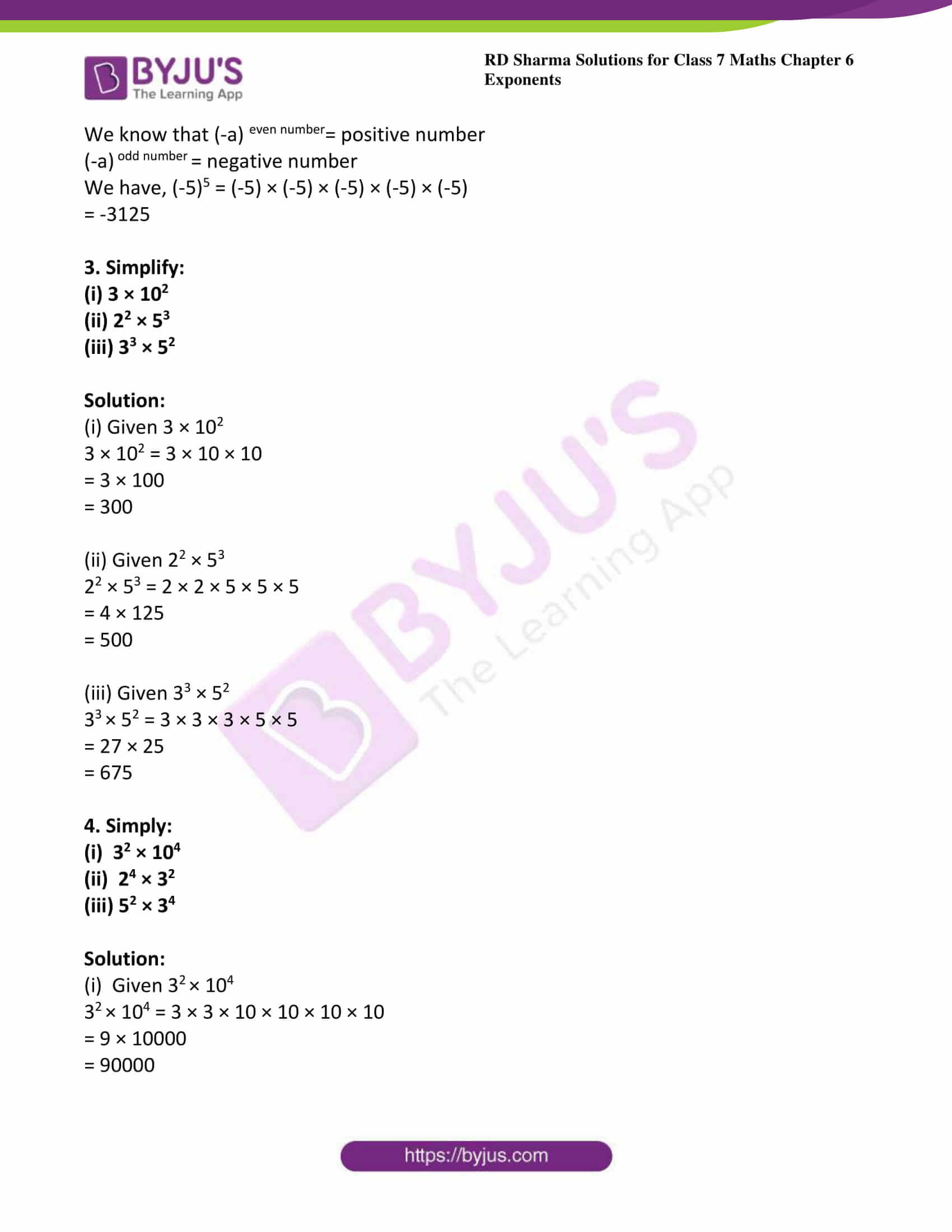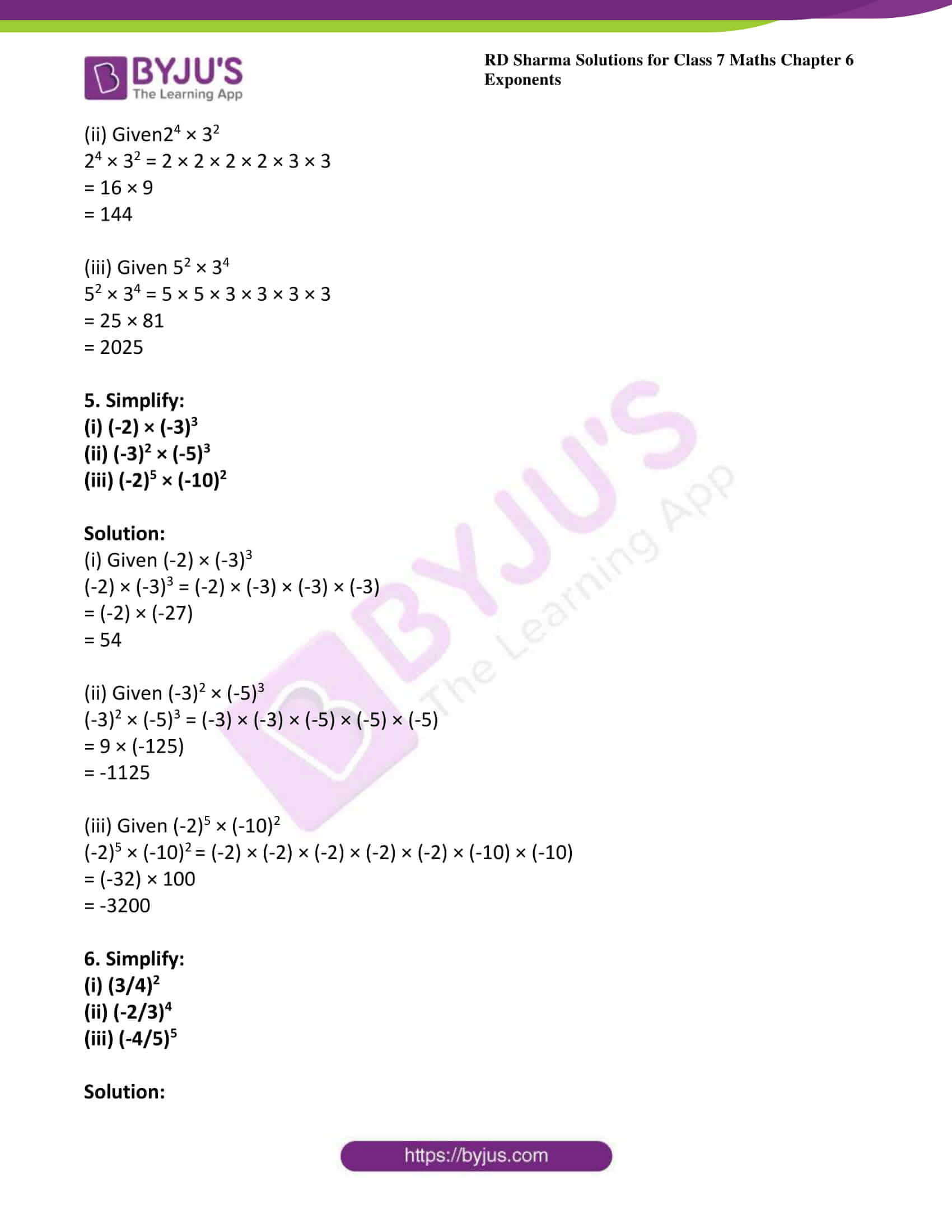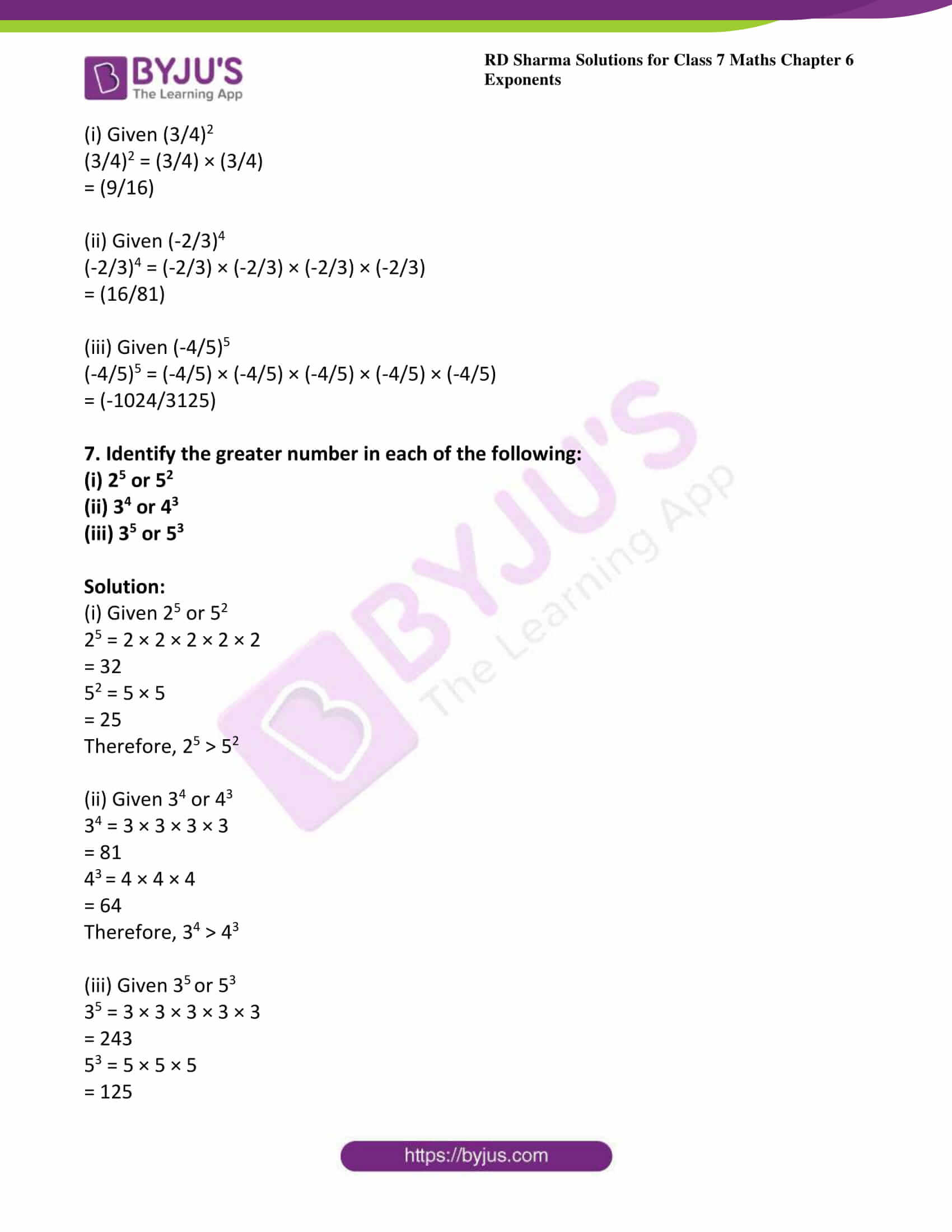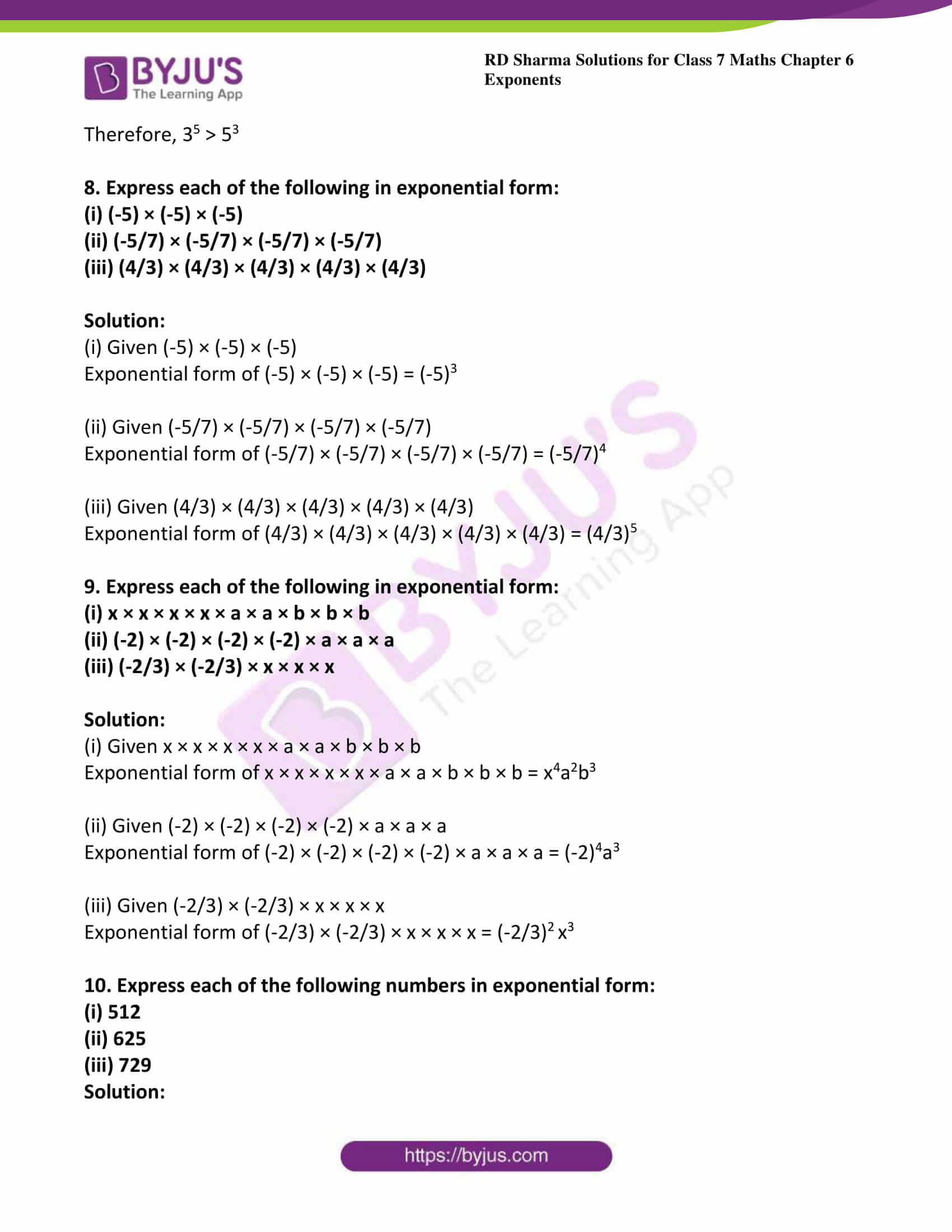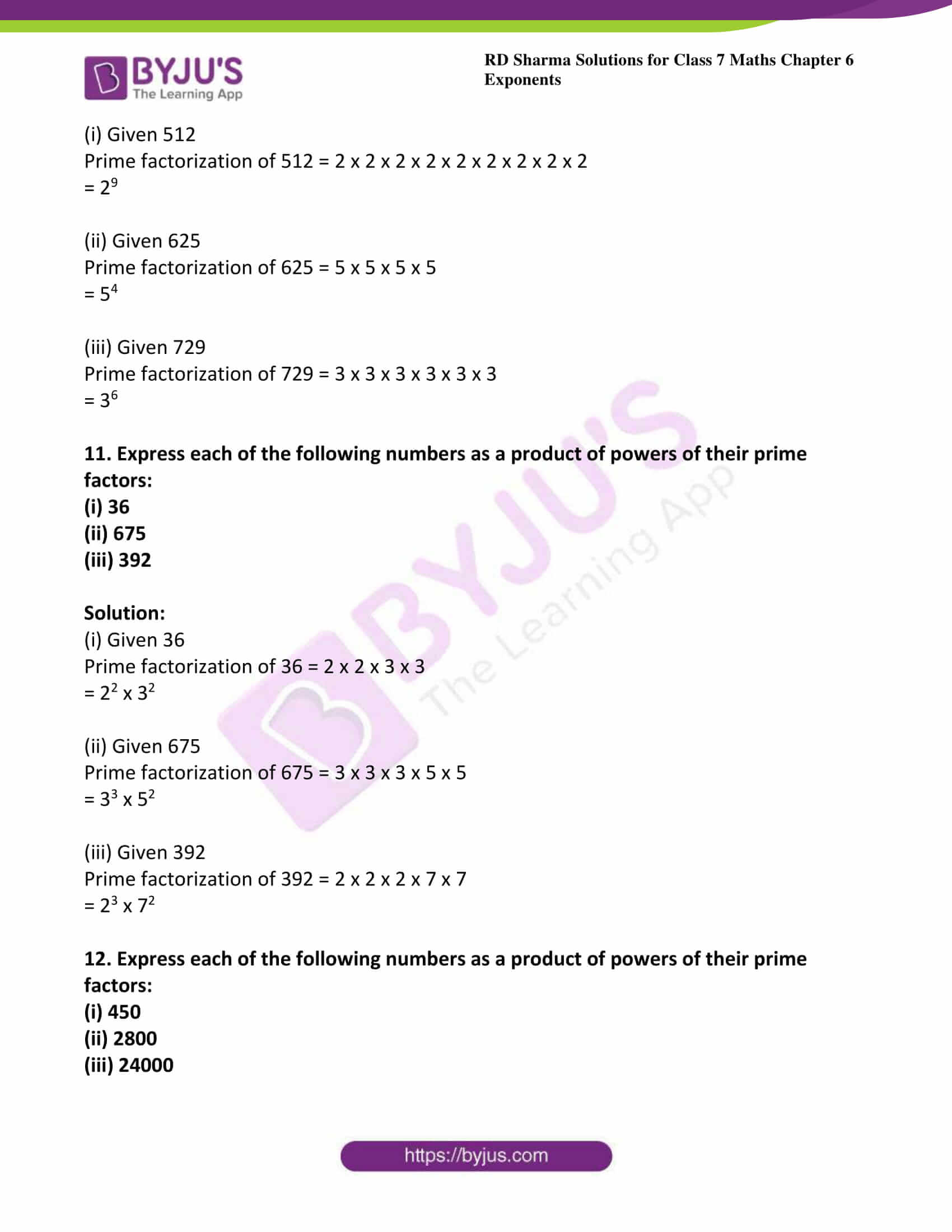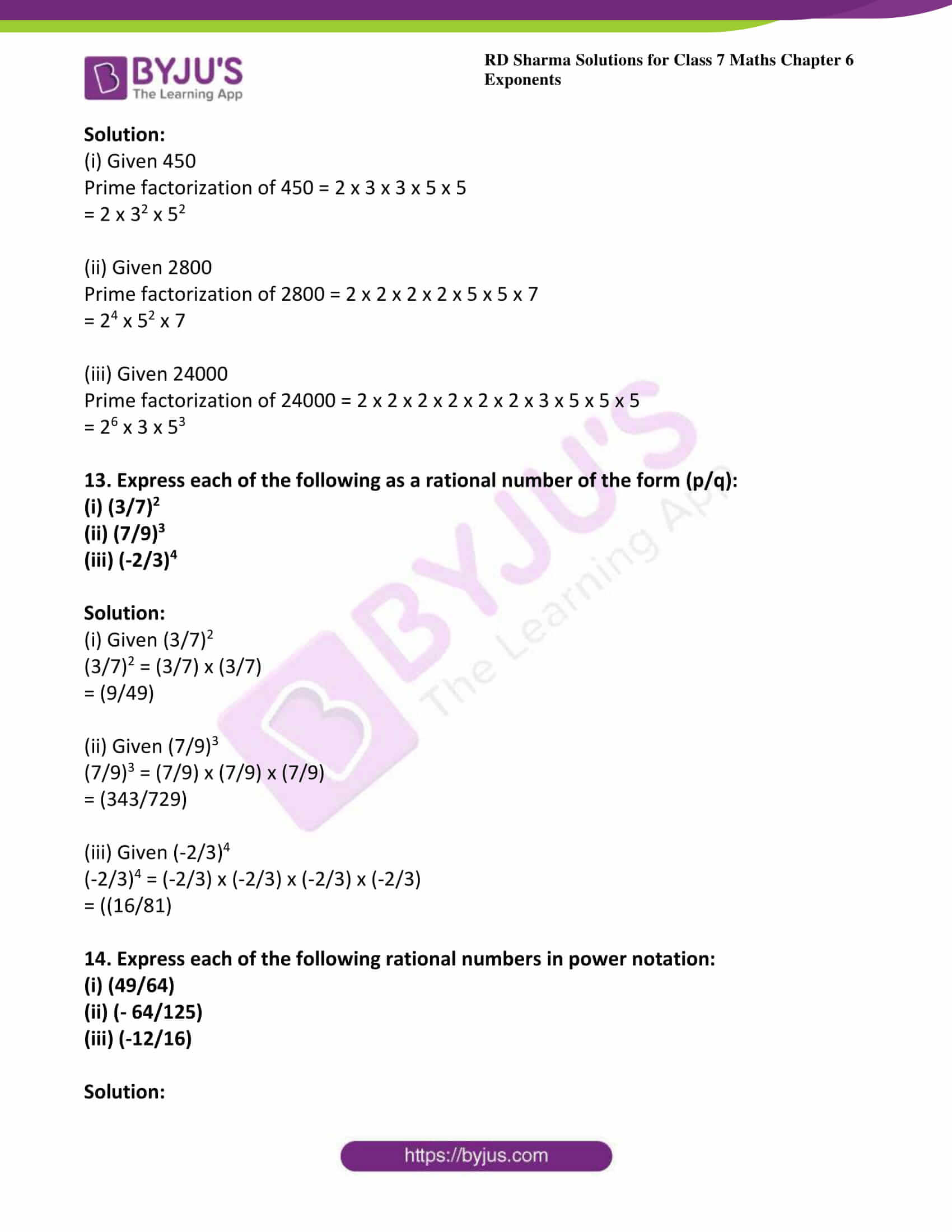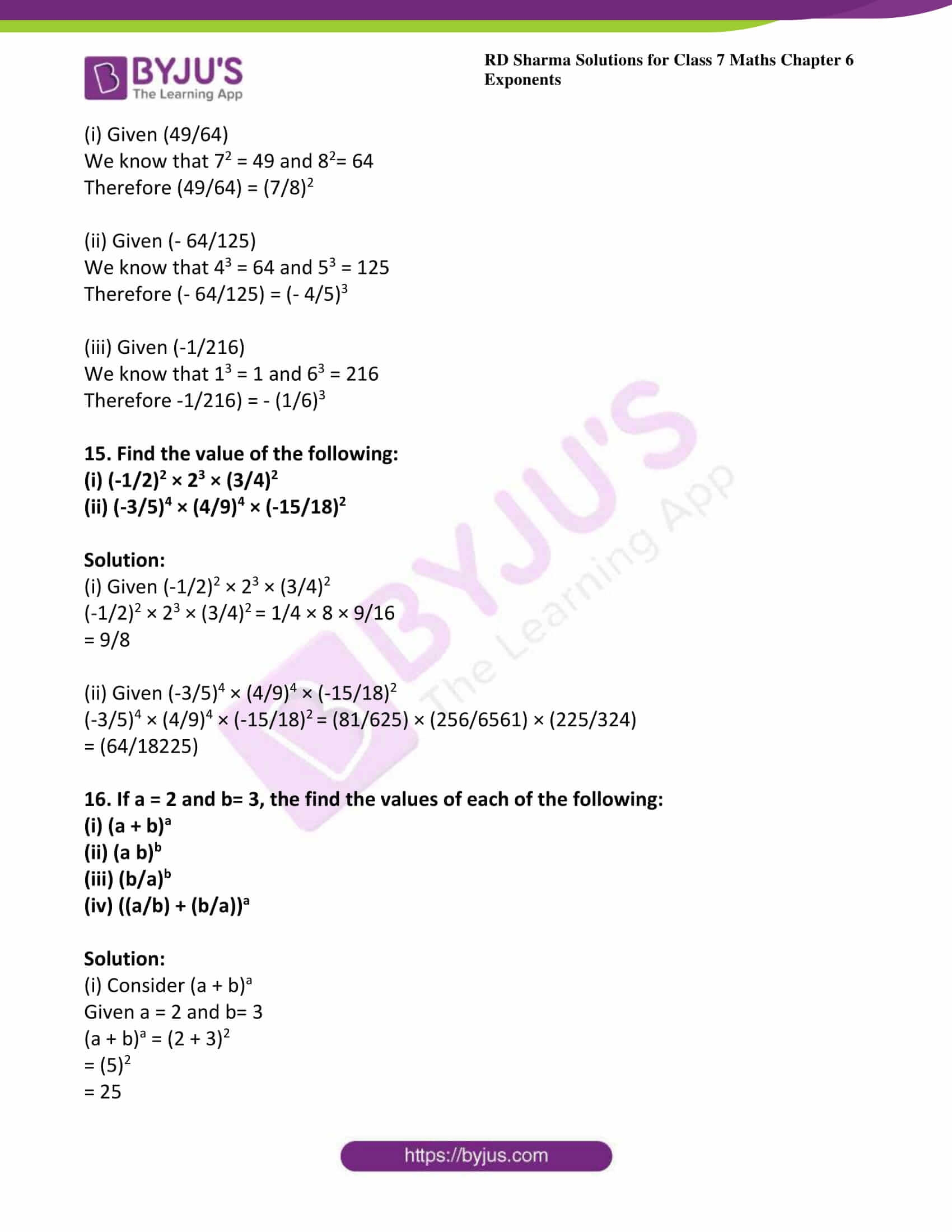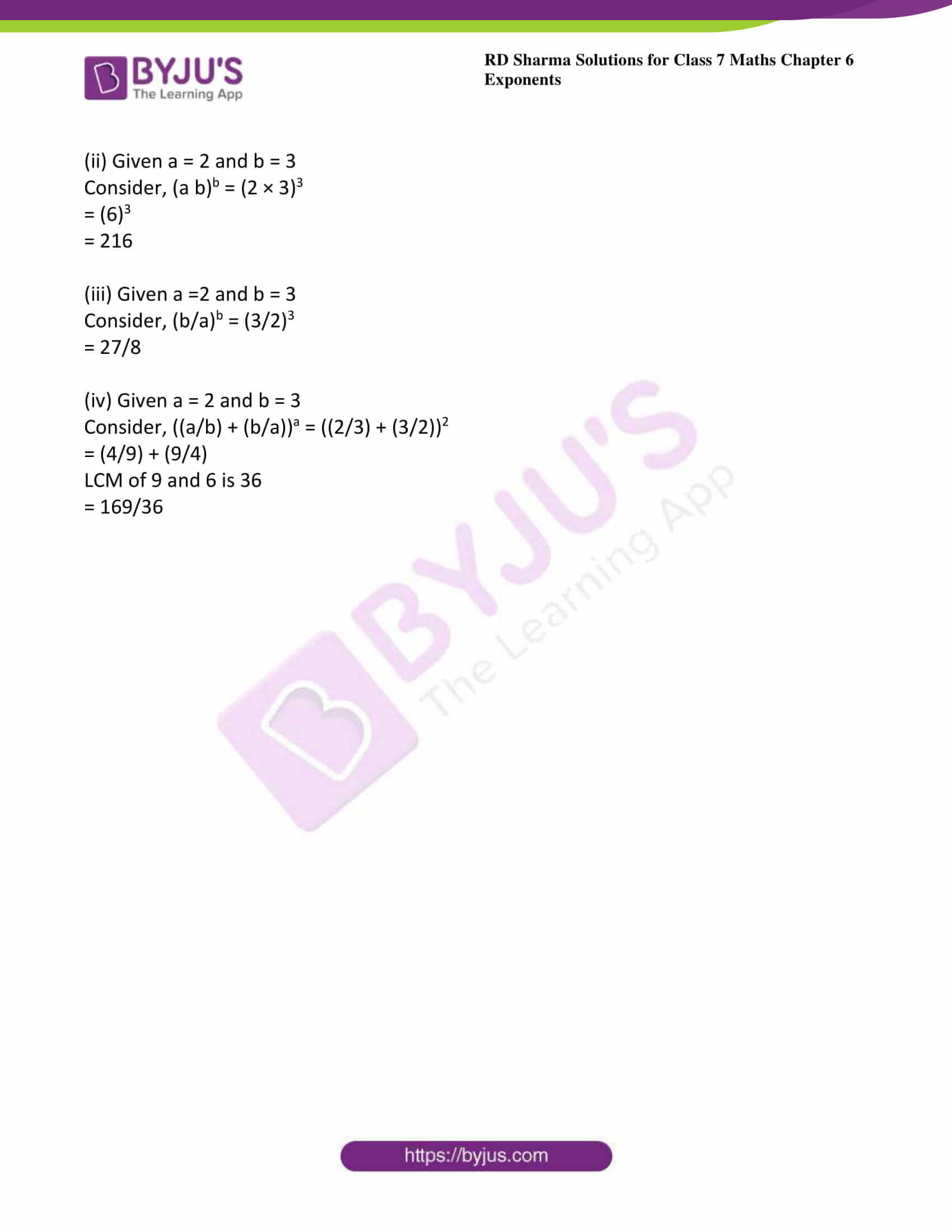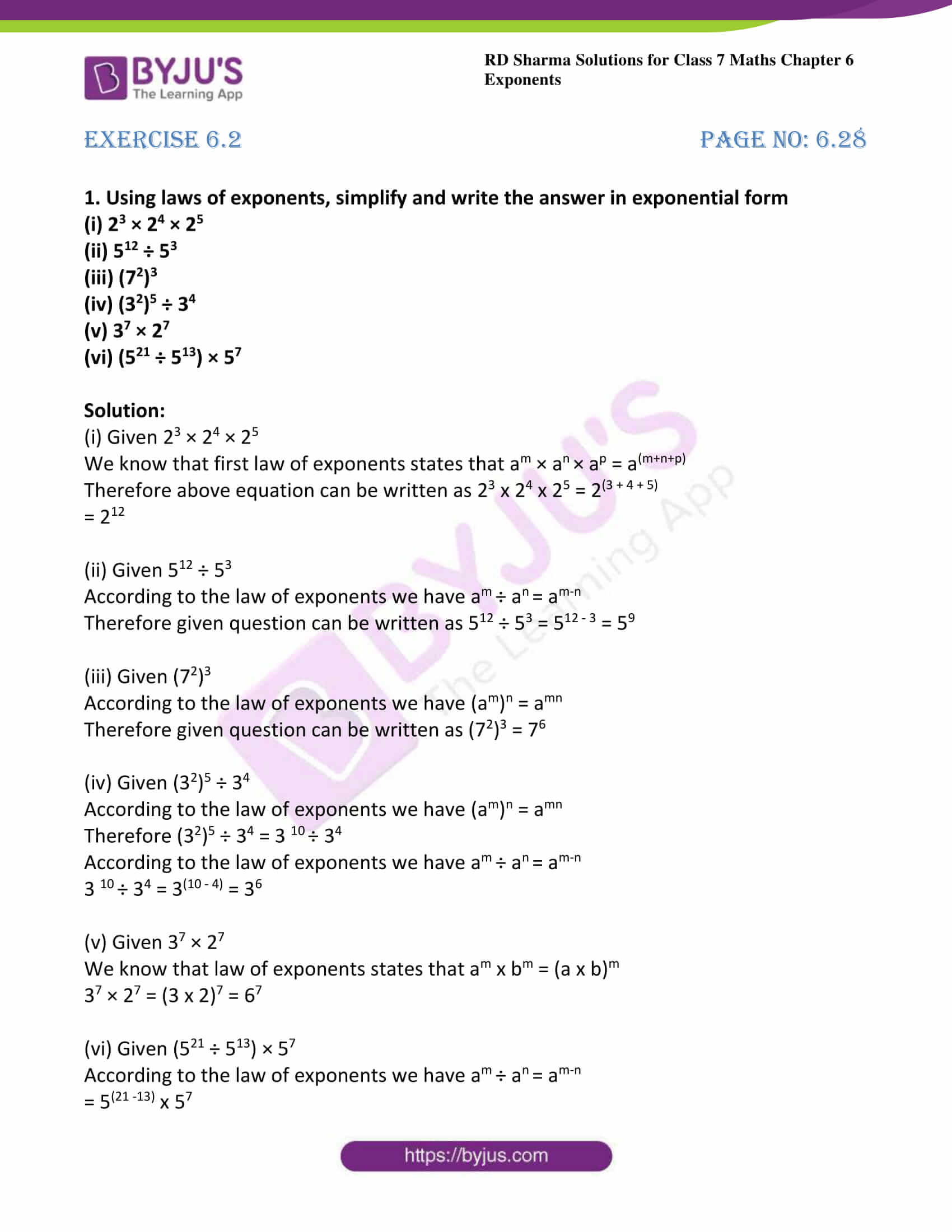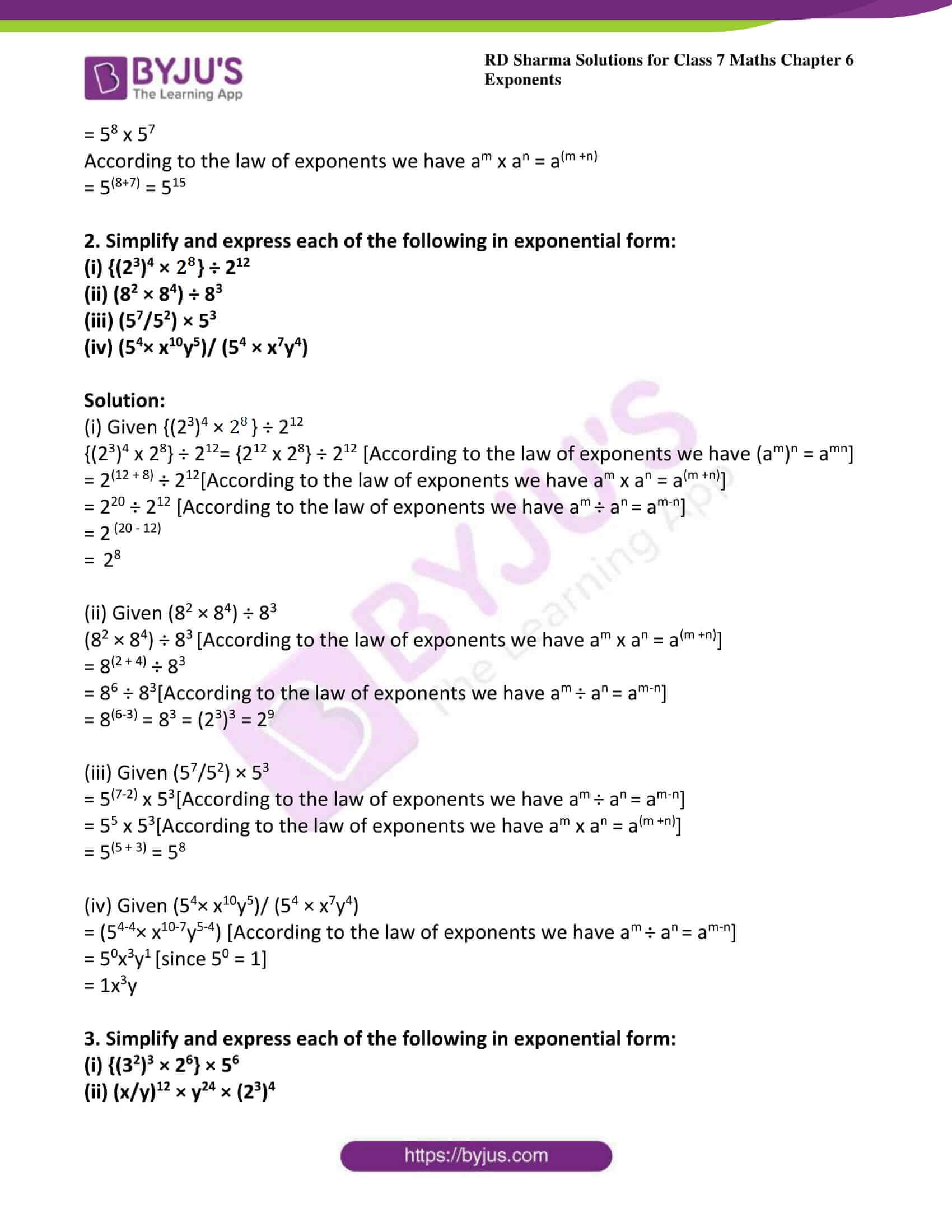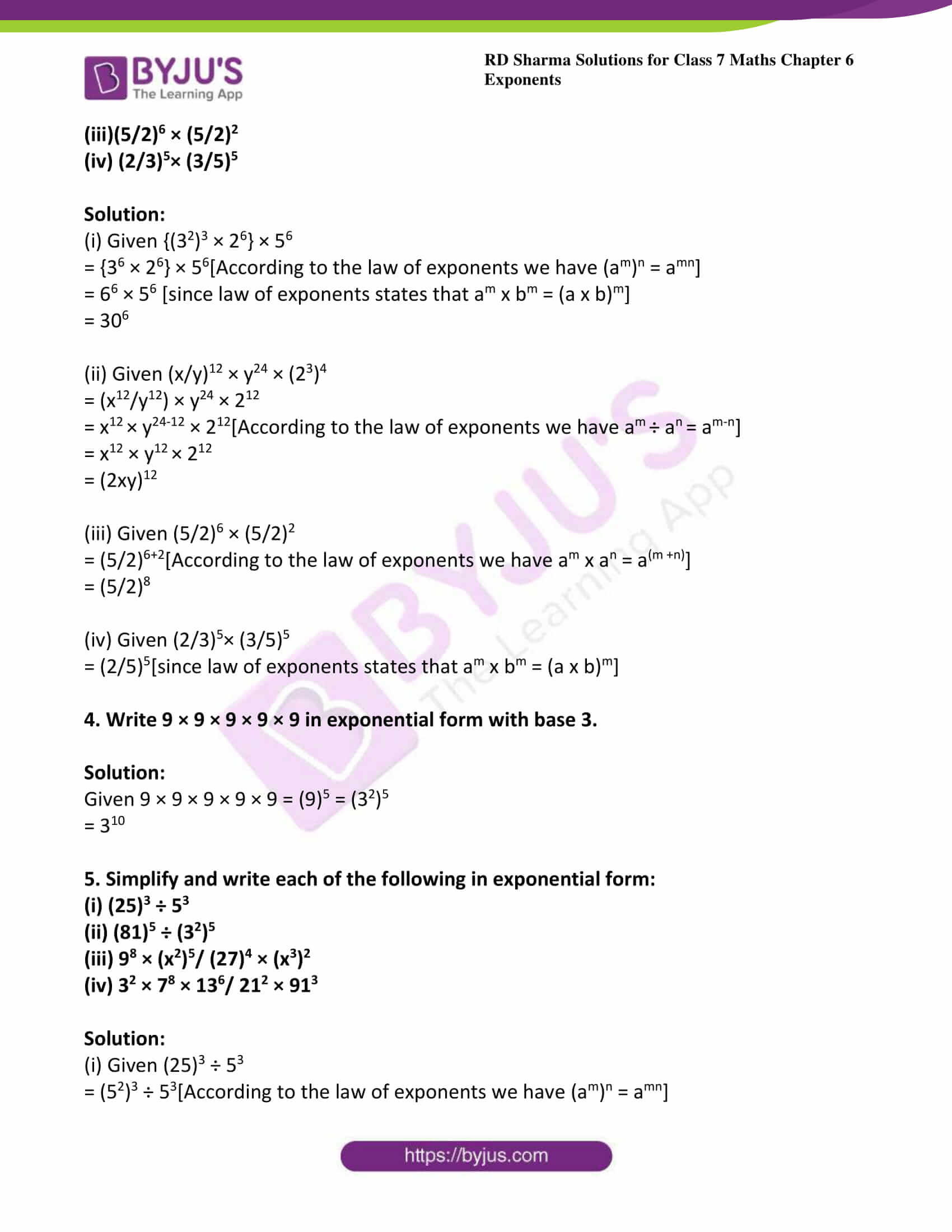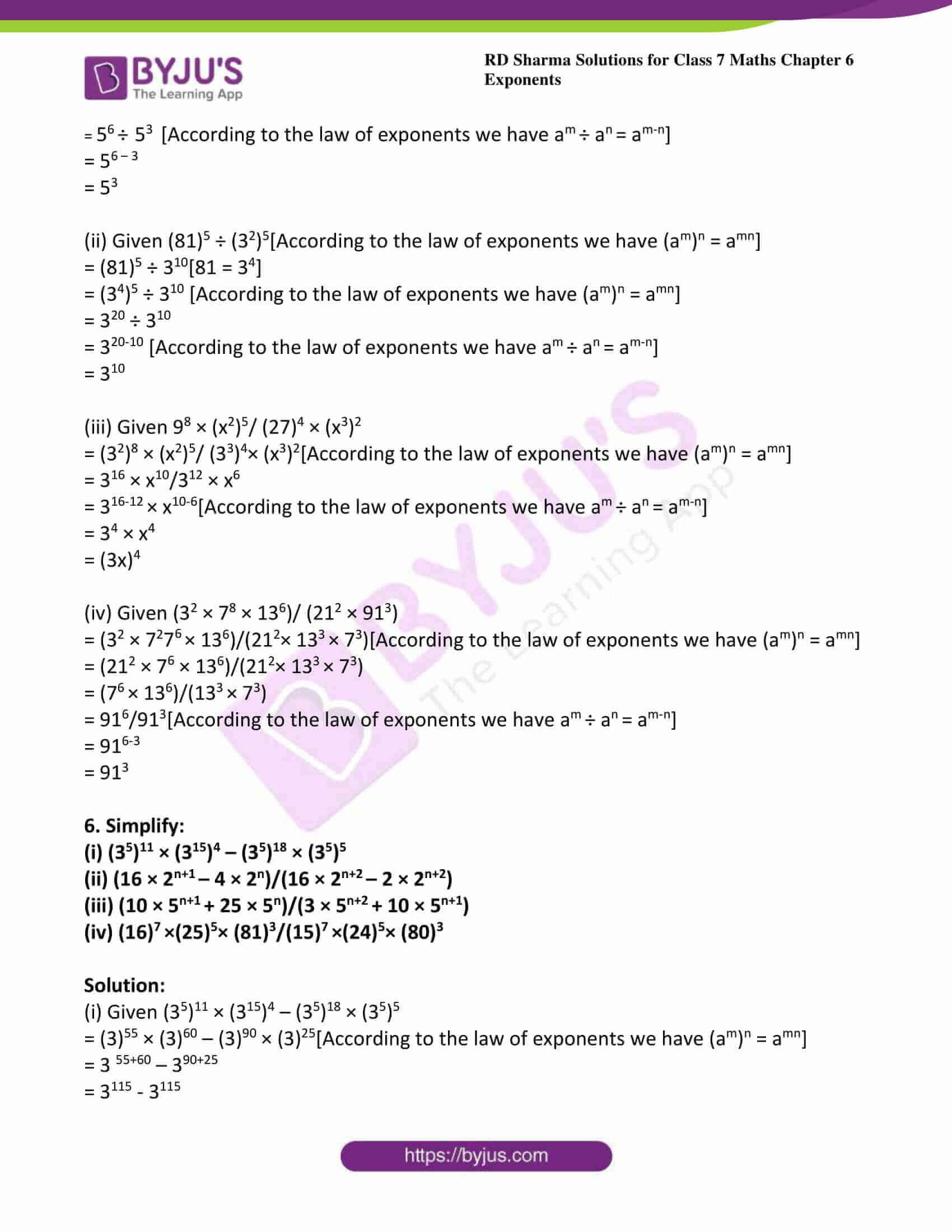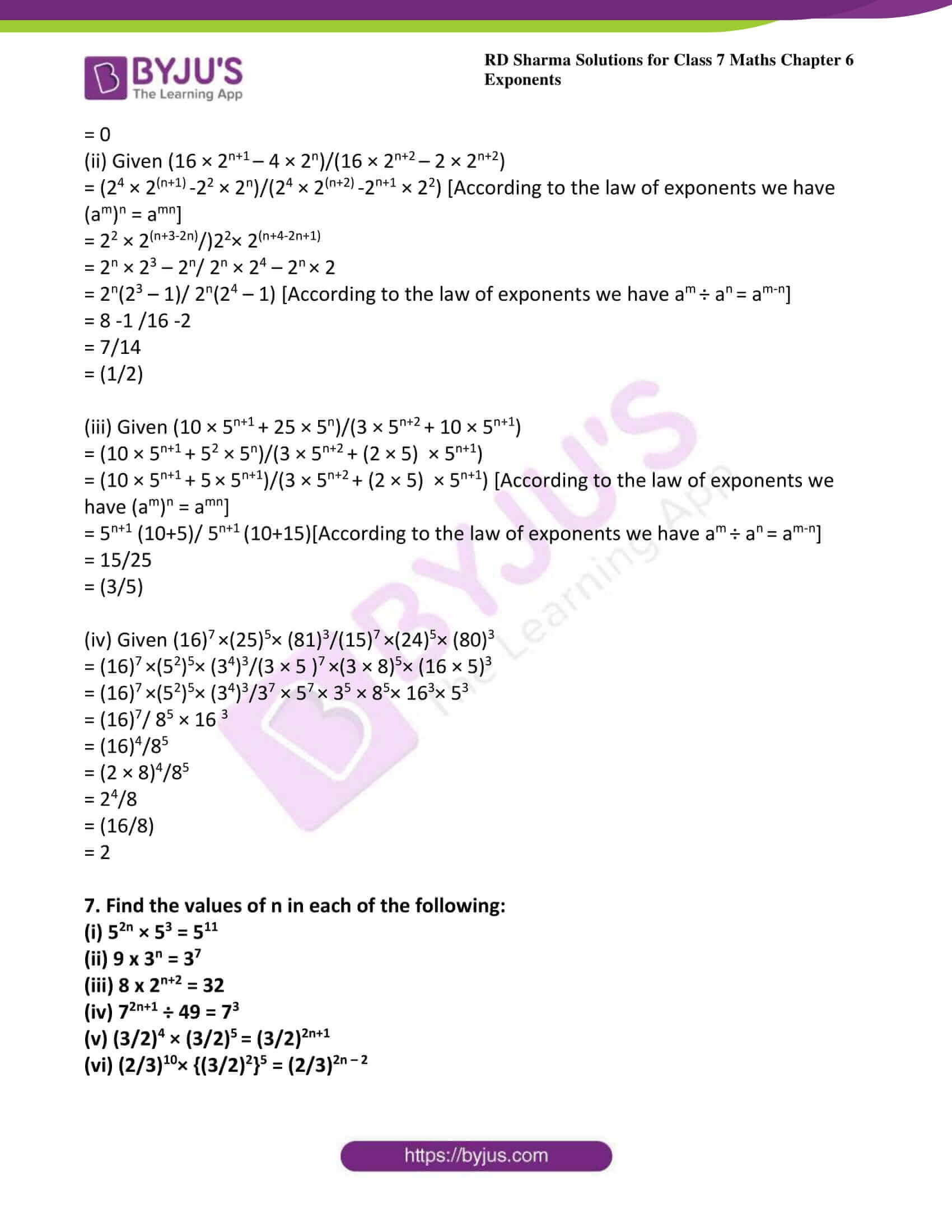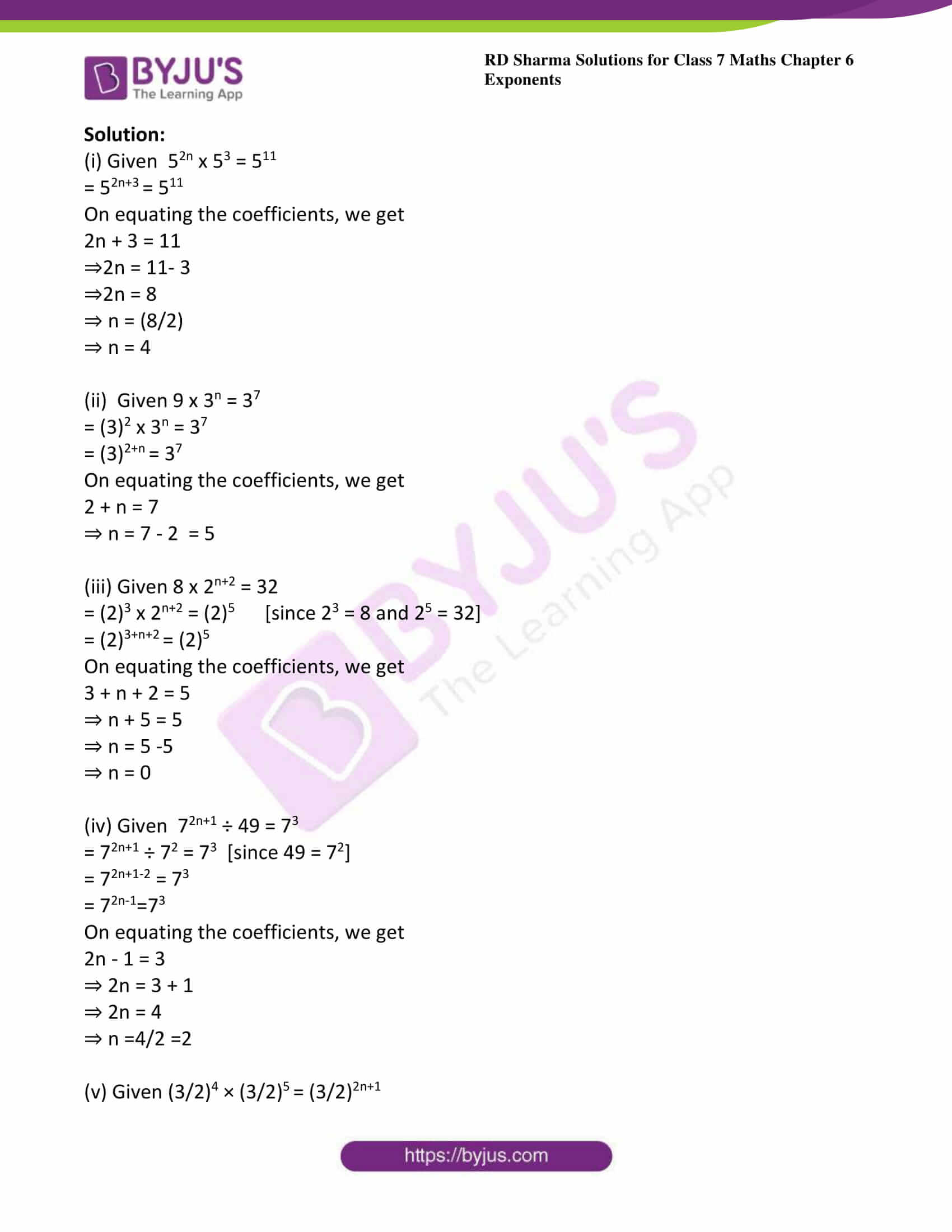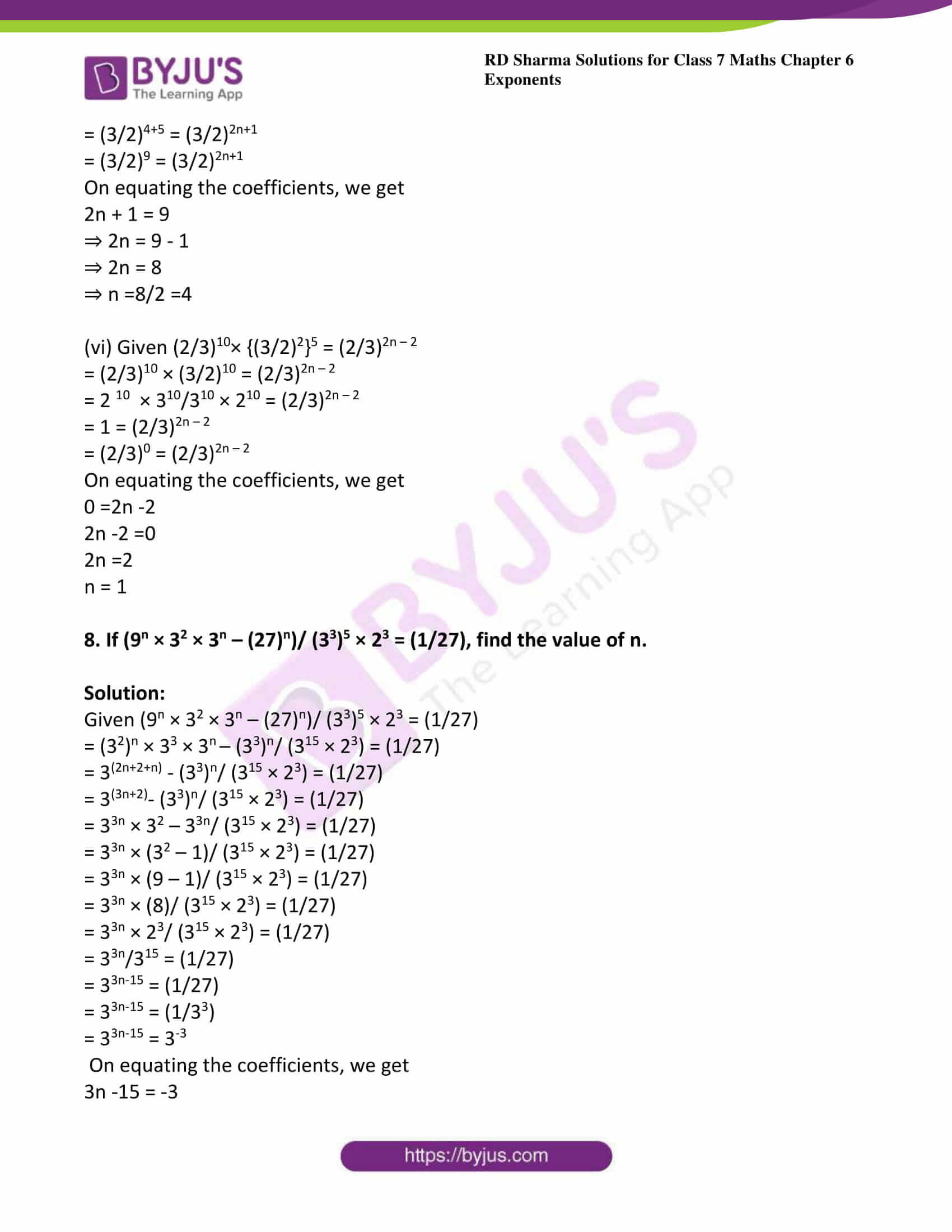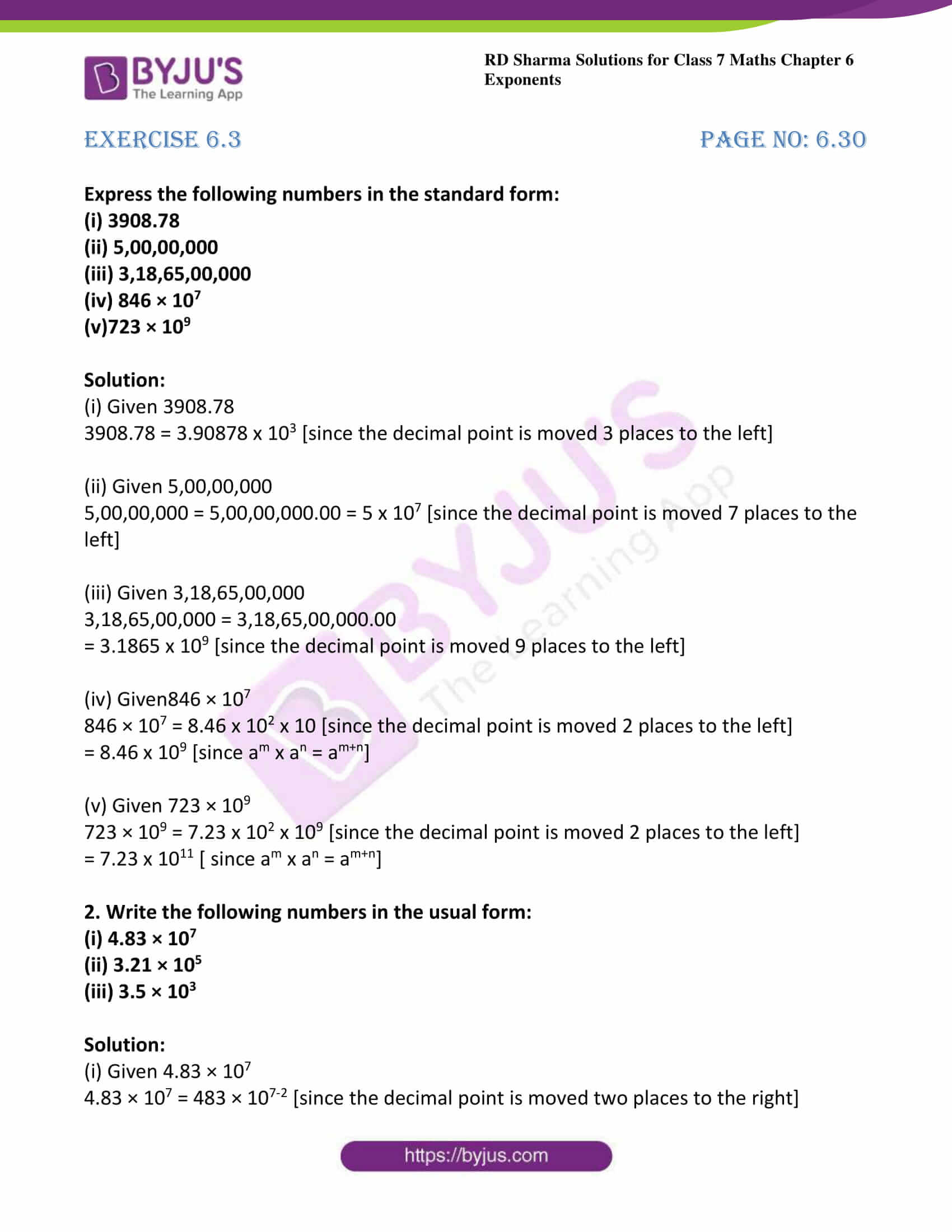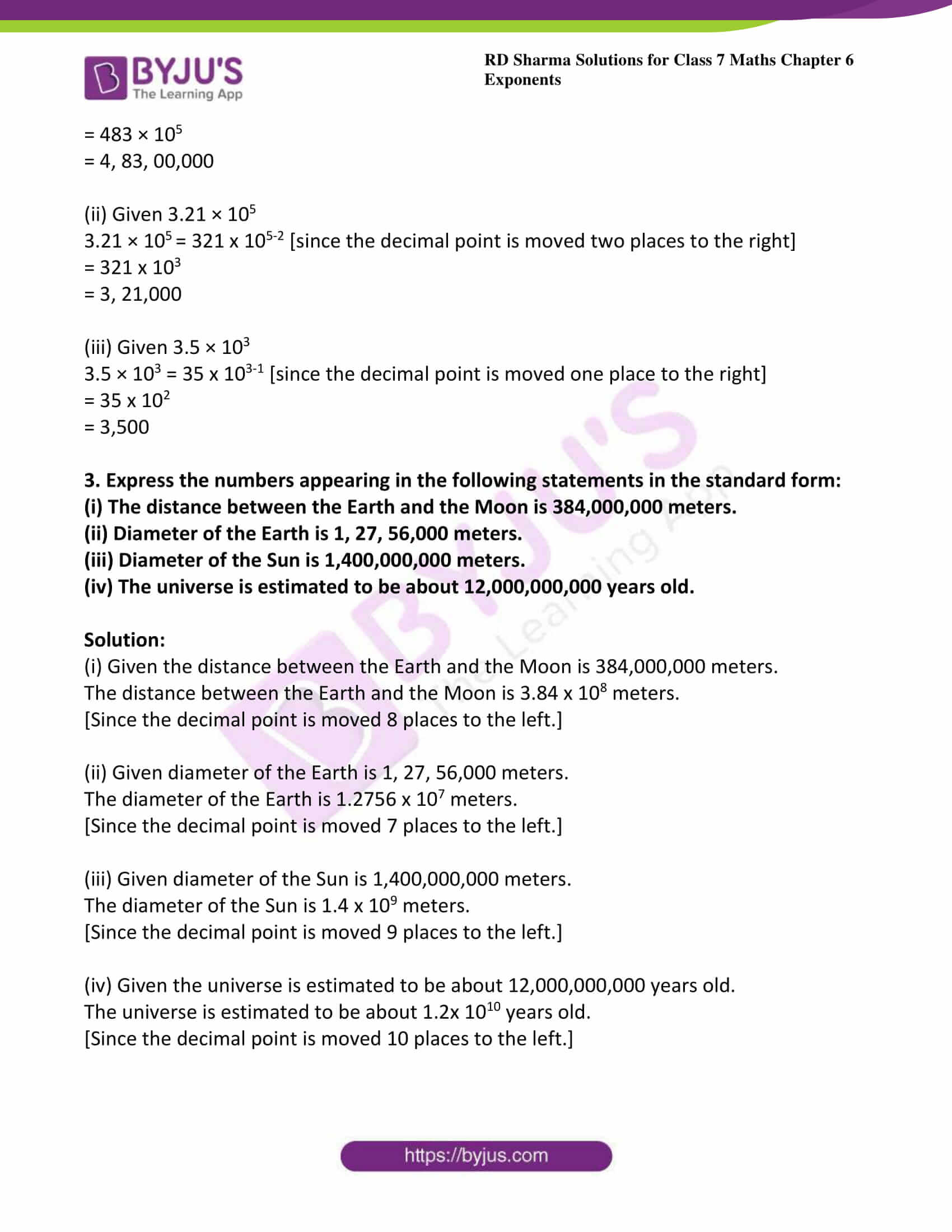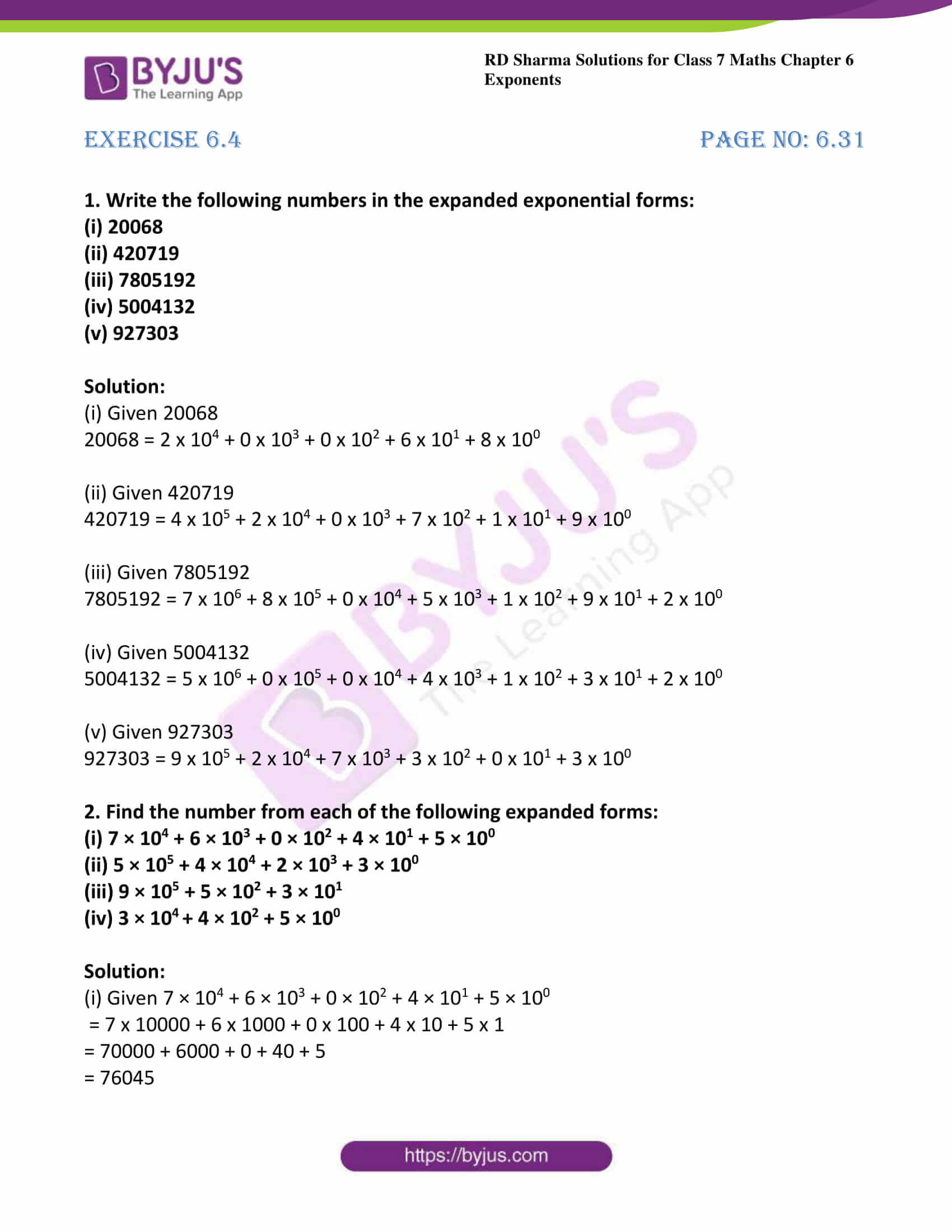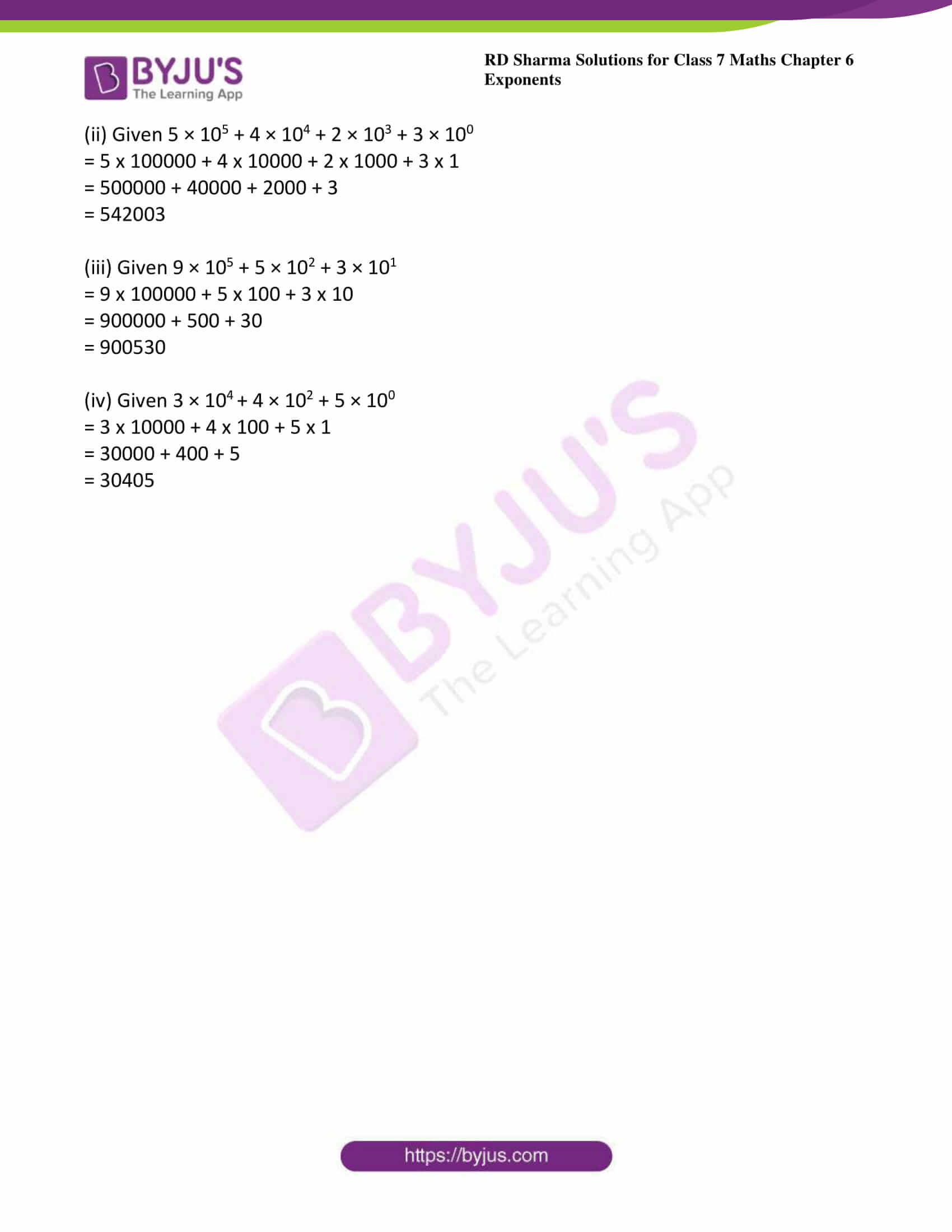### Access answers to Maths RD Sharma Solutions For Class 7 Chapter 6 – Exponents

Exercise 6.1 Page No: 6.12

1. Find the values of each of the following:

(i) 132

(ii) 73

(iii) 34

Solution:

(i) Given 132

132 = 13 × 13 =169

(ii) Given 73

73 = 7 × 7 × 7 = 343

(iii) Given 34

34 = 3 × 3 × 3 × 3

= 81

2. Find the value of each of the following:

(i) (-7)2

(ii) (-3)4

(iii)  (-5)5

Solution:

(i) Given (-7)2

We know that (-a) even number= positive number

(-a) odd number = negative number

We have, (-7)2 = (-7) × (-7)

= 49

(ii) Given (-3)4

We know that (-a) even number= positive number

(-a) odd number = negative number

We have, (-3)4 = (-3) × (-3) × (-3) × (-3)

= 81

(iii) Given (-5)5

We know that (-a) even number= positive number

(-a) odd number = negative number

We have, (-5)5 = (-5) × (-5) × (-5) × (-5) × (-5)

= -3125

3. Simplify:

(i) 3 × 102

(ii) 22 × 53

(iii) 33 × 52

Solution:

(i) Given 3 × 102

3 × 102 = 3 × 10 × 10

= 3 × 100

= 300

(ii) Given 22 × 53

22 × 53 = 2 × 2 × 5 × 5 × 5

= 4 × 125

= 500

(iii) Given 33 × 52

3× 52 = 3 × 3 × 3 × 5 × 5

= 27 × 25

= 675

4. Simply:

(i)  32 × 104

(ii)  24 × 32

(iii) 52 × 34

Solution:

(i)  Given 3× 104

3× 104 = 3 × 3 × 10 × 10 × 10 × 10

= 9 × 10000

= 90000

(ii) Given24 × 32

24 × 32 = 2 × 2 × 2 × 2 × 3 × 3

= 16 × 9

= 144

(iii) Given 52 × 34

52 × 34 = 5 × 5 × 3 × 3 × 3 × 3

= 25 × 81

= 2025

5. Simplify:

(i) (-2) × (-3)3

(ii) (-3)2 × (-5)3

(iii) (-2)5 × (-10)2

Solution:

(i) Given (-2) × (-3)3

(-2) × (-3)3 = (-2) × (-3) × (-3) × (-3)

= (-2) × (-27)

= 54

(ii) Given (-3)2 × (-5)3

(-3)2 × (-5)3 = (-3) × (-3) × (-5) × (-5) × (-5)

= 9 × (-125)

= -1125

(iii) Given (-2)5 × (-10)

(-2)5 × (-10)= (-2) × (-2) × (-2) × (-2) × (-2) × (-10) × (-10)

= (-32) × 100

= -3200

6. Simplify:

(i) (3/4)2

(ii) (-2/3)4

(iii) (-4/5)5

Solution:

(i) Given (3/4)2

(3/4)2 = (3/4) × (3/4)

= (9/16)

(ii) Given (-2/3)4

(-2/3)4 = (-2/3) × (-2/3) × (-2/3) × (-2/3)

= (16/81)

(iii) Given (-4/5)5

(-4/5)5 = (-4/5) × (-4/5) × (-4/5) × (-4/5) × (-4/5)

= (-1024/3125)

7. Identify the greater number in each of the following:

(i) 25 or 52

(ii) 34 or 43

(iii) 35 or 53

Solution:

(i) Given 25 or 52

25 = 2 × 2 × 2 × 2 × 2

= 32

52 = 5 × 5

= 25

Therefore, 25 > 52

(ii) Given 34 or 43

34 = 3 × 3 × 3 × 3

= 81

4= 4 × 4 × 4

= 64

Therefore, 34 > 43

(iii) Given 3or 53

35 = 3 × 3 × 3 × 3 × 3

= 243

53 = 5 × 5 × 5

= 125

Therefore, 35 > 53

8. Express each of the following in exponential form:

(i) (-5) × (-5) × (-5)

(ii) (-5/7) × (-5/7) × (-5/7) × (-5/7)

(iii) (4/3) × (4/3) × (4/3) × (4/3) × (4/3)

Solution:

(i) Given (-5) × (-5) × (-5)

Exponential form of (-5) × (-5) × (-5) = (-5)3

(ii) Given (-5/7) × (-5/7) × (-5/7) × (-5/7)

Exponential form of (-5/7) × (-5/7) × (-5/7) × (-5/7) = (-5/7)4

(iii) Given (4/3) × (4/3) × (4/3) × (4/3) × (4/3)

Exponential form of (4/3) × (4/3) × (4/3) × (4/3) × (4/3) = (4/3)5

9. Express each of the following in exponential form:

(i) x × x × x × x × a × a × b × b × b

(ii) (-2) × (-2) × (-2) × (-2) × a × a × a

(iii) (-2/3) × (-2/3) × x × x × x

Solution:

(i) Given x × x × x × x × a × a × b × b × b

Exponential form of x × x × x × x × a × a × b × b × b = x4a2b3

(ii) Given (-2) × (-2) × (-2) × (-2) × a × a × a

Exponential form of (-2) × (-2) × (-2) × (-2) × a × a × a = (-2)4a3

(iii) Given (-2/3) × (-2/3) × x × x × x

Exponential form of (-2/3) × (-2/3) × x × x × x = (-2/3)x3

10. Express each of the following numbers in exponential form:

(i) 512

(ii) 625

(iii) 729

Solution:

(i) Given 512

Prime factorization of 512 = 2 x 2 x 2 x 2 x 2 x 2 x 2 x 2 x 2

= 29

(ii) Given 625

Prime factorization of 625 = 5 x 5 x 5 x 5

= 54

(iii) Given 729

Prime factorization of 729 = 3 x 3 x 3 x 3 x 3 x 3

= 36

11. Express each of the following numbers as a product of powers of their prime factors:
(i) 36
(ii) 675
(iii) 392

Solution:

(i) Given 36

Prime factorization of 36 = 2 x 2 x 3 x 3

= 22 x 32

(ii) Given 675

Prime factorization of 675 = 3 x 3 x 3 x 5 x 5

= 33 x 52

(iii) Given 392

Prime factorization of 392 = 2 x 2 x 2 x 7 x 7

= 23 x 72

12. Express each of the following numbers as a product of powers of their prime factors:
(i) 450
(ii) 2800
(iii) 24000

Solution:

(i) Given 450

Prime factorization of 450 = 2 x 3 x 3 x 5 x 5

= 2 x 32 x 52

(ii) Given 2800

Prime factorization of 2800 = 2 x 2 x 2 x 2 x 5 x 5 x 7

= 24 x 52 x 7

(iii) Given 24000

Prime factorization of 24000 = 2 x 2 x 2 x 2 x 2 x 2 x 3 x 5 x 5 x 5

= 26 x 3 x 53

13. Express each of the following as a rational number of the form (p/q):

(i) (3/7)2

(ii) (7/9)3

(iii) (-2/3)4

Solution:

(i) Given (3/7)2

(3/7)2 = (3/7) x (3/7)

= (9/49)

(ii) Given (7/9)3

(7/9)3 = (7/9) x (7/9) x (7/9)

= (343/729)

(iii) Given (-2/3)4

(-2/3)4 = (-2/3) x (-2/3) x (-2/3) x (-2/3)

= ((16/81)

14. Express each of the following rational numbers in power notation:

(i) (49/64)

(ii) (- 64/125)

(iii) (-12/16)

Solution:

(i) Given (49/64)

We know that 72 = 49 and 82= 64

Therefore (49/64) = (7/8)2

(ii) Given (- 64/125)

We know that 43 = 64 and 53 = 125

Therefore (- 64/125) = (- 4/5)3

(iii) Given (-1/216)

We know that 13 = 1 and 63 = 216

Therefore -1/216) = – (1/6)3

15. Find the value of the following:

(i) (-1/2)2 × 23 × (3/4)2

(ii) (-3/5)4 × (4/9)4 × (-15/18)2

Solution:

(i) Given (-1/2)2 × 23 × (3/4)2

(-1/2)2 × 23 × (3/4)= 1/4 × 8 × 9/16

= 9/8

(ii) Given (-3/5)4 × (4/9)4 × (-15/18)

(-3/5)4 × (4/9)4 × (-15/18)= (81/625) × (256/6561) × (225/324)

= (64/18225)

16. If a = 2 and b= 3, the find the values of each of the following:

(i) (a + b)a

(ii) (a b)b

(iii) (b/a)b

(iv) ((a/b) + (b/a))a

Solution:

(i) Consider (a + b)a

Given a = 2 and b= 3

(a + b)a = (2 + 3)2

= (5)2

= 25

(ii) Given a = 2 and b = 3

Consider, (a b)b = (2 × 3)3

= (6)3

= 216

(iii) Given a =2 and b = 3

Consider, (b/a)b = (3/2)3

= 27/8

(iv) Given a = 2 and b = 3

Consider, ((a/b) + (b/a))a = ((2/3) + (3/2))2

= (4/9) + (9/4)

LCM of 9 and 6 is 36

= 169/36

Exercise 6.2 Page No: 6.28

1. Using laws of exponents, simplify and write the answer in exponential form

(i) 23 × 24 × 25

(ii) 512 ÷ 53

(iii) (72)3

(iv) (32)5 ÷ 34

(v) 37 × 27

(vi) (521 ÷ 513) × 57

Solution:

(i) Given 23 × 24 × 25

We know that first law of exponents states that am × a× ap = a(m+n+p)

Therefore above equation can be written as 23 x 24 x 25 = 2(3 + 4 + 5)

= 212

(ii) Given 512 ÷ 53

According to the law of exponents we have a÷ a= am-n

Therefore given question can be written as 512 ÷ 53 = 512 – 3 = 59

(iii) Given (72)3

According to the law of exponents we have (am)n = amn

Therefore given question can be written as (72)3 = 76

(iv) Given (32)5 ÷ 34

According to the law of exponents we have (am)n = amn

Therefore (32)5 ÷ 34 = 3 10 ÷ 34

According to the law of exponents we have a÷ a= am-n

3 10 ÷ 34 = 3(10 – 4) = 36

(v) Given 37 × 27

We know that law of exponents states that am x bm = (a x b)m

37 × 27 = (3 x 2)7 = 67

(vi) Given (521 ÷ 513) × 57

According to the law of exponents we have a÷ a= am-n

= 5(21 -13) x 57

= 58 x 57

According to the law of exponents we have am x an = a(m +n)

= 5(8+7) = 515

2. Simplify and express each of the following in exponential form:

(i) {(23)4 × 28} ÷ 212

(ii) (82 × 84) ÷ 83

(iii) (57/52) × 53

(iv) (54× x10y5)/ (54 × x7y4)

Solution:

(i) Given {(23)4 × 28} ÷ 212

{(23)4 x 28} ÷ 212= {212 x 28} ÷ 212 [According to the law of exponents we have (am)n = amn]

= 2(12 + 8) ÷ 212[According to the law of exponents we have am x an = a(m +n)]

= 220 ÷ 212 [According to the law of exponents we have a÷ a= am-n]

= 2 (20 – 12)

=  28

(ii) Given (82 × 84) ÷ 83

(82 × 84) ÷ 83 [According to the law of exponents we have am x an = a(m +n)]

= 8(2 + 4) ÷ 83

= 86 ÷ 83[According to the law of exponents we have a÷ a= am-n]

= 8(6-3) = 83 = (23)3 = 29

(iii) Given (57/52) × 53

= 5(7-2) x 53[According to the law of exponents we have a÷ a= am-n]

= 55 x 53[According to the law of exponents we have am x an = a(m +n)]

= 5(5 + 3) = 58

(iv) Given (54× x10y5)/ (54 × x7y4)

= (54-4× x10-7y5-4) [According to the law of exponents we have a÷ a= am-n]

= 50x3y1 [since 50 = 1]

= 1x3y

3. Simplify and express each of the following in exponential form:

(i) {(32)3 × 26} × 56

(ii) (x/y)12 × y24 × (23)4

(iii)(5/2)6 × (5/2)2

(iv) (2/3)5× (3/5)5

Solution:

(i) Given {(32)3 × 26} × 56

= {36 × 26} × 56[According to the law of exponents we have (am)n = amn]

= 66 × 56 [since law of exponents states that am x bm = (a x b)m]

= 306

(ii) Given (x/y)12 × y24 × (23)4

= (x12/y12) × y24 × 212

= x12 × y24-12 × 212[According to the law of exponents we have a÷ a= am-n]

= x12 × y12 × 212

= (2xy)12

(iii) Given (5/2)6 × (5/2)2

= (5/2)6+2[According to the law of exponents we have am x an = a(m +n)]

= (5/2)8

(iv) Given (2/3)5× (3/5)5

= (2/5)5[since law of exponents states that am x bm = (a x b)m]

4. Write 9 × 9 × 9 × 9 × 9 in exponential form with base 3.

Solution:

Given 9 × 9 × 9 × 9 × 9 = (9)5 = (32)5

= 310

5. Simplify and write each of the following in exponential form:

(i) (25)3 ÷ 53

(ii) (81)5 ÷ (32)5

(iii) 98 × (x2)5/ (27)4 × (x3)2

(iv) 32 × 78 × 136/ 212 × 913

Solution:

(i) Given (25)3 ÷ 53

= (52)3 ÷ 53[According to the law of exponents we have (am)n = amn]

= 56 ÷ 53 [According to the law of exponents we have a÷ a= am-n]

= 56 – 3

= 53

(ii) Given (81)5 ÷ (32)5[According to the law of exponents we have (am)n = amn]

= (81)5 ÷ 310[81 = 34]

= (34)5 ÷ 310 [According to the law of exponents we have (am)n = amn]

= 320 ÷ 310

= 320-10 [According to the law of exponents we have a÷ a= am-n]

= 310

(iii) Given 98 × (x2)5/ (27)4 × (x3)2

= (32)8 × (x2)5/ (33)4× (x3)2[According to the law of exponents we have (am)n = amn]

= 316 × x10/312 × x6

= 316-12 × x10-6[According to the law of exponents we have a÷ a= am-n]

= 34 × x4

= (3x)4

(iv) Given (32 × 78 × 136)/ (212 × 913)

= (32 × 7276 × 136)/(212× 133 × 73)[According to the law of exponents we have (am)n = amn]

= (212 × 76 × 136)/(212× 133 × 73)

= (76 × 136)/(133 × 73)

= 916/913[According to the law of exponents we have a÷ a= am-n]

= 916-3

= 913

6. Simplify:

(i) (35)11 × (315)4 – (35)18 × (35)5

(ii) (16 × 2n+1 – 4 × 2n)/(16 × 2n+2 – 2 × 2n+2)

(iii) (10 × 5n+1 + 25 × 5n)/(3 × 5n+2 + 10 × 5n+1)

(iv) (16)7 ×(25)5× (81)3/(15)7 ×(24)5× (80)3

Solution:

(i) Given (35)11 × (315)4 – (35)18 × (35)5

= (3)55 × (3)60 – (3)90 × (3)25[According to the law of exponents we have (am)n = amn]

= 3 55+60 – 390+25

= 3115 – 3115

= 0

(ii) Given (16 × 2n+1 – 4 × 2n)/(16 × 2n+2 – 2 × 2n+2)

= (24 × 2(n+1) -22 × 2n)/(24 × 2(n+2) -2n+1 × 22) [According to the law of exponents we have (am)n = amn]

= 22 × 2(n+3-2n)/)22× 2(n+4-2n+1)

= 2n × 23 – 2n/ 2n × 24 – 2n × 2

= 2n(23 – 1)/ 2n(24 – 1) [According to the law of exponents we have a÷ a= am-n]

= 8 -1 /16 -2

= 7/14

= (1/2)

(iii) Given (10 × 5n+1 + 25 × 5n)/(3 × 5n+2 + 10 × 5n+1)

= (10 × 5n+1 + 52 × 5n)/(3 × 5n+2 + (2 × 5) × 5n+1)

= (10 × 5n+1 + 5 × 5n+1)/(3 × 5n+2 + (2 × 5) × 5n+1) [According to the law of exponents we have (am)n = amn]

= 5n+1 (10+5)/ 5n+1 (10+15)[According to the law of exponents we have a÷ a= am-n]

= 15/25

= (3/5)

(iv) Given (16)7 ×(25)5× (81)3/(15)7 ×(24)5× (80)3

= (16)7 ×(52)5× (34)3/(3 × 5 )7 ×(3 × 8)5× (16 × 5)3

= (16)7 ×(52)5× (34)3/37 × 57 × 35 × 85× 163× 53

= (16)7/ 85 × 16 3

= (16)4/85

= (2 × 8)4/85

= 24/8

= (16/8)

= 2

7. Find the values of n in each of the following:

(i) 52n × 53 = 511

(ii) 9 x 3n = 37

(iii) 8 x 2n+2 = 32

(iv) 72n+1 ÷ 49 = 73

(v) (3/2)4 × (3/2)5 = (3/2)2n+1

(vi) (2/3)10× {(3/2)2}5 = (2/3)2n – 2

Solution:

(i) Given 52n x 53 = 511

= 52n+3 = 511

On equating the coefficients, we get

2n + 3 = 11

⇒2n = 11- 3

⇒2n = 8

⇒ n = (8/2)

⇒ n = 4

(ii)  Given 9 x 3n = 37

= (3)2 x 3n = 37

= (3)2+n = 37

On equating the coefficients, we get

2 + n = 7

⇒ n = 7 – 2  = 5

(iii) Given 8 x 2n+2 = 32

= (2)3 x 2n+2 = (2)5      [since 23 = 8 and 25 = 32]

= (2)3+n+2 = (2)5

On equating the coefficients, we get

3 + n + 2 = 5

⇒ n + 5 = 5

⇒ n = 5 -5

⇒ n = 0

(iv) Given  72n+1 ÷ 49 = 73

= 72n+1 ÷ 72 = 73  [since 49 = 72]

= 72n+1-2 = 73

= 72n-1=73

On equating the coefficients, we get

2n – 1 = 3

⇒ 2n = 3 + 1

⇒ 2n = 4

⇒ n =4/2 =2

(v) Given (3/2)4 × (3/2)5 = (3/2)2n+1

= (3/2)4+5 = (3/2)2n+1

= (3/2)9 = (3/2)2n+1

On equating the coefficients, we get

2n + 1 = 9

⇒ 2n = 9 – 1

⇒ 2n = 8

⇒ n =8/2 =4

(vi) Given (2/3)10× {(3/2)2}5 = (2/3)2n – 2

= (2/3)10 × (3/2)10 = (2/3)2n – 2

= 2 10 × 310/310 × 210 = (2/3)2n – 2

= 1 = (2/3)2n – 2

= (2/3)0 = (2/3)2n – 2

On equating the coefficients, we get

0 =2n -2

2n -2 =0

2n =2

n = 1

8. If (9n × 32 × 3n – (27)n)/ (33)5 × 23 = (1/27), find the value of n.

Solution:

Given (9n × 32 × 3n – (27)n)/ (33)5 × 23 = (1/27)

= (32)n × 33 × 3n – (33)n/ (315 × 23) = (1/27)

= 3(2n+2+n) – (33)n/ (315 × 23) = (1/27)

= 3(3n+2)– (33)n/ (315 × 23) = (1/27)

= 33n × 32 – 33n/ (315 × 23) = (1/27)

= 33n × (32 – 1)/ (315 × 23) = (1/27)

= 33n × (9 – 1)/ (315 × 23) = (1/27)

= 33n × (8)/ (315 × 23) = (1/27)

= 33n × 23/ (315 × 23) = (1/27)

= 33n/315 = (1/27)

= 33n-15 = (1/27)

= 33n-15 = (1/33)

= 33n-15 = 3-3

On equating the coefficients, we get

3n -15 = -3

⇒ 3n = -3 + 15

⇒ 3n = 12

⇒ n = 12/3 = 4

Exercise 6.3 Page No: 6.30

Express the following numbers in the standard form:
(i) 3908.78
(ii) 5,00,00,000
(iii) 3,18,65,00,000
(iv) 846 × 107
(v)723 × 109

Solution:

(i) Given 3908.78

3908.78 = 3.90878 x 103 [since the decimal point is moved 3 places to the left]

(ii) Given 5,00,00,000

5,00,00,000 = 5,00,00,000.00 = 5 x 107 [since the decimal point is moved 7 places to the left]

(iii) Given 3,18,65,00,000

3,18,65,00,000 = 3,18,65,00,000.00

= 3.1865 x 109 [since the decimal point is moved 9 places to the left]

(iv) Given846 × 107

846 × 107 = 8.46 x 102 x 10 [since the decimal point is moved 2 places to the left]

= 8.46 x 109 [since am x an = am+n]

(v) Given 723 × 109

723 × 109 = 7.23 x 102 x 109 [since the decimal point is moved 2 places to the left]

= 7.23 x 1011 [ since am x an = am+n]

2. Write the following numbers in the usual form:
(i) 4.83 × 107
(ii) 3.21 × 105
(iii) 3.5 × 103

Solution:

(i) Given 4.83 × 107

4.83 × 107 = 483 × 107-2 [since the decimal point is moved two places to the right]

= 483 × 105

= 4, 83, 00,000

(ii) Given 3.21 × 10

3.21 × 10= 321 x 105-2 [since the decimal point is moved two places to the right]

= 321 x 103

= 3, 21,000

(iii) Given 3.5 × 103

3.5 × 103 = 35 x 103-1 [since the decimal point is moved one place to the right]

= 35 x 102

= 3,500

3. Express the numbers appearing in the following statements in the standard form:

(i) The distance between the Earth and the Moon is 384,000,000 meters.
(ii) Diameter of the Earth is 1, 27, 56,000 meters.
(iii) Diameter of the Sun is 1,400,000,000 meters.
(iv) The universe is estimated to be about 12,000,000,000 years old.

Solution:

(i) Given the distance between the Earth and the Moon is 384,000,000 meters.

The distance between the Earth and the Moon is 3.84 x 108 meters.

[Since the decimal point is moved 8 places to the left.]

(ii) Given diameter of the Earth is 1, 27, 56,000 meters.

The diameter of the Earth is 1.2756 x 107 meters.

[Since the decimal point is moved 7 places to the left.]

(iii) Given diameter of the Sun is 1,400,000,000 meters.

The diameter of the Sun is 1.4 x 109 meters.

[Since the decimal point is moved 9 places to the left.]

(iv) Given the universe is estimated to be about 12,000,000,000 years old.

The universe is estimated to be about 1.2x 1010 years old.

[Since the decimal point is moved 10 places to the left.]

Exercise 6.4 Page No: 6.31

1. Write the following numbers in the expanded exponential forms:
(i) 20068
(ii) 420719
(iii) 7805192
(iv) 5004132
(v) 927303

Solution:

(i) Given 20068

20068 = 2 x 104 + 0 x 103 + 0 x 102 + 6 x 101 + 8 x 100

(ii) Given 420719

420719 = 4 x 105 + 2 x 104 + 0 x 103 + 7 x 102 + 1 x 101 + 9 x 100

(iii) Given 7805192

7805192 = 7 x 106 + 8 x 105 + 0 x 104 + 5 x 103 + 1 x 102 + 9 x 101 + 2 x 100

(iv) Given 5004132

5004132 = 5 x 106 + 0 x 105 + 0 x 104 + 4 x 103 + 1 x 102 + 3 x 101 + 2 x 100

(v) Given 927303

927303 = 9 x 105 + 2 x 104 + 7 x 103 + 3 x 102 + 0 x 101 + 3 x 100

2. Find the number from each of the following expanded forms:
(i) 7 × 104 + 6 × 103 + 0 × 102 + 4 × 101 + 5 × 100
(ii) 5 × 105 + 4 × 104 + 2 × 103 + 3 × 100
(iii) 9 × 105 + 5 × 102 + 3 × 101
(iv) 3 × 10+ 4 × 102 + 5 × 100

Solution:

(i) Given 7 × 104 + 6 × 103 + 0 × 102 + 4 × 101 + 5 × 100

= 7 x 10000 + 6 x 1000 + 0 x 100 + 4 x 10 + 5 x 1

= 70000 + 6000 + 0 + 40 + 5

= 76045

(ii) Given 5 × 105 + 4 × 104 + 2 × 103 + 3 × 100

= 5 x 100000 + 4 x 10000 + 2 x 1000 + 3 x 1

= 500000 + 40000 + 2000 + 3

= 542003

(iii) Given 9 × 105 + 5 × 102 + 3 × 101

= 9 x 100000 + 5 x 100 + 3 x 10

= 900000 + 500 + 30

= 900530

(iv) Given 3 × 10+ 4 × 102 + 5 × 100

= 3 x 10000 + 4 x 100 + 5 x 1

= 30000 + 400 + 5

= 30405